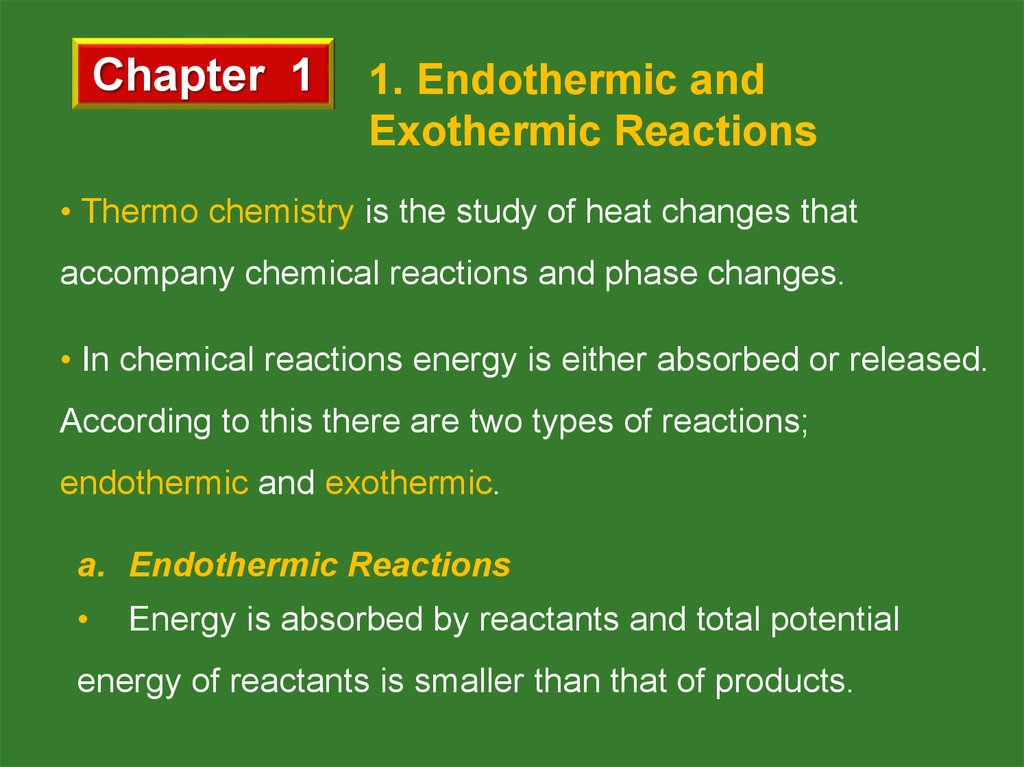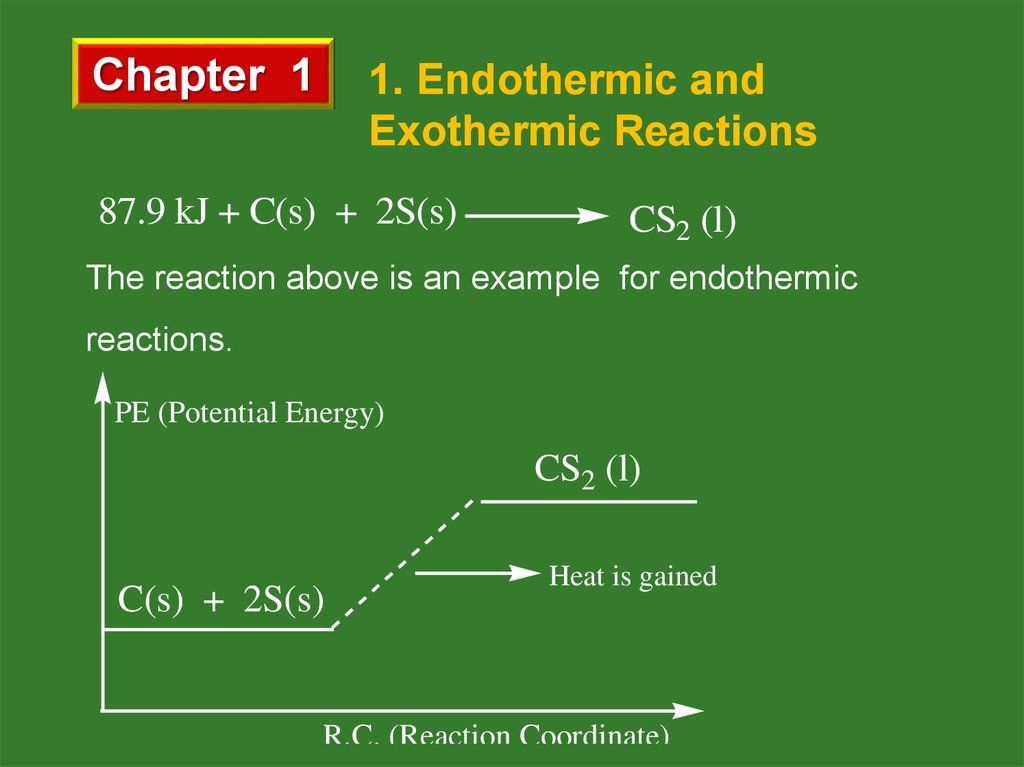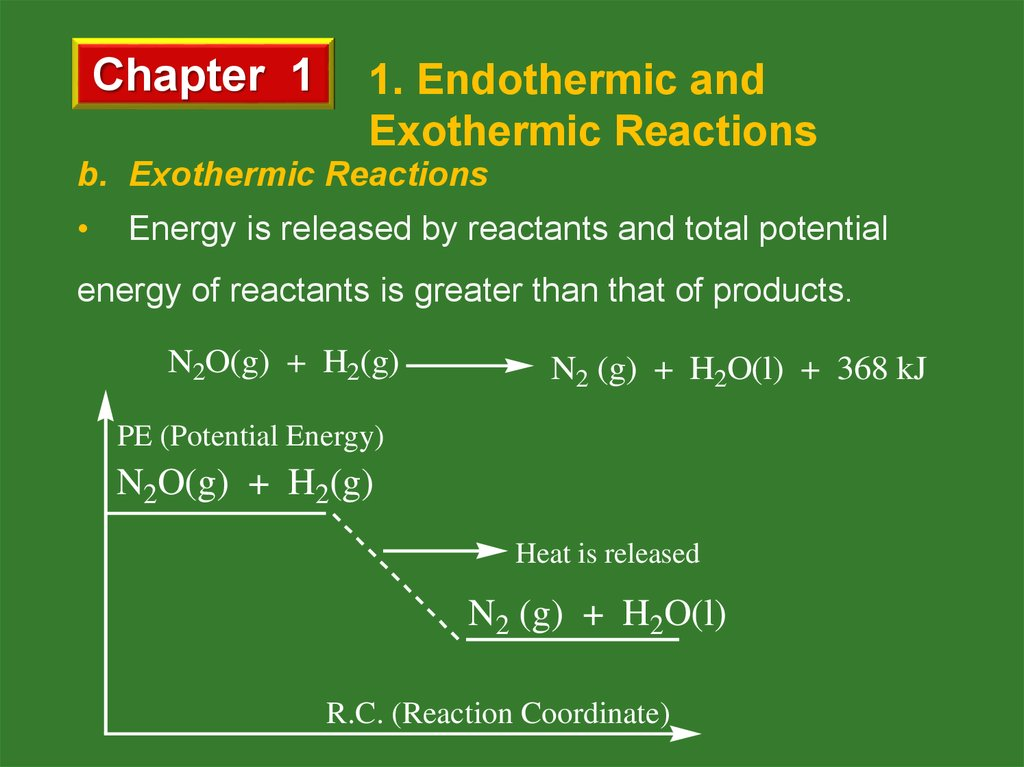# Chemical reactions and heat. (Chapter 1)

## 1. Slayt 1

Chapter 1
1. Endothermic and
Exothermic Reactions
• Thermo chemistry is the study of heat changes that
accompany chemical reactions and phase changes.
• In chemical reactions energy is either absorbed or released.
According to this there are two types of reactions;
endothermic and exothermic.
a. Endothermic Reactions
Energy is absorbed by reactants and total potential
energy of reactants is smaller than that of products.

## 2. Slayt 2

Chapter 1
1. Endothermic and
Exothermic Reactions
87.9 kJ + C(s) + 2S(s)
CS2 (l)
The reaction above is an example for endothermic
reactions.
PE (Potential Energy)
CS2 (l)
C(s) + 2S(s)
Heat is gained
R.C. (Reaction Coordinate)

## 3. Slayt 3

Chapter 1
1. Endothermic and
Exothermic Reactions
b. Exothermic Reactions
Energy is released by reactants and total potential
energy of reactants is greater than that of products.
N2O(g) + H2(g)
N2 (g) + H2O(l) + 368 kJ
PE (Potential Energy)
N2O(g) + H2(g)
Heat is released
N2 (g) + H2O(l)
R.C. (Reaction Coordinate)

## 4. Slayt 4

Chapter 1
1. Endothermic and
Exothermic Reactions

## 5. Slayt 5

Chapter 1
1. Endothermic and
Exothermic Reactions

## 6. Slayt 6

Chapter 1
2. Enthalpy Change of
Reactions (ΔH)
Enthalpy (H) is the heat content of a substance at
constant pressure.
The change in enthalpy for a reaction is called the
enthalpy of reaction (∆H).
ΔH = ΣHproducts
ΣHreactants
If ΣHproducts > ΣHreactants, then ∆H > 0 so the reaction is
endothermic. Similarly,
If ΣHproducts < ΣHreactants, then ∆H < 0 so the reaction is
exothermic.

## 7. Slayt 7

Chapter 1
2. Enthalpy Change of
Reactions (ΔH)

## 8. Slayt 8

Chapter 1
2. Enthalpy Change of
Reactions (ΔH)
Example 1
C(s) + O2(g)
CO2(g) + 393.5 kJ
ΔH = - 393.5 kJ < 0 then the reaction
is exothermic.
PE (Potential Energy)
C(s) + O2(g)
Heat is
released
H = - 393.5 kJ
CO2(g)
R.C. (Reaction Coordinate)

## 9. Slayt 9

Chapter 1
2. Enthalpy Change of
Reactions (ΔH)
Example 2
H2O(l) + 242 kJ
H2(g) + O2(g)
ΔH = 242 kJ > 0 then the reaction is
endothermic.
PE (Potential Energy)
H2(g) + O2 (g)
Heat is gained
H = 242 kJ
H2O(l)
R.C. (Reaction Coordinate)

## 10. Slayt 10

Chapter 1
2. Enthalpy Change of
Reactions (ΔH)
Standard Heat of Formation (ΔHof)
The heat change when 1 mole compound is produced
from its elements in their most stable states (under 1 atm
pressure and at 25oC is called as standard heat of
formation, and shown by ΔHof .
ΔHof of the free atoms (K, Fe, Na, S, P, Cu…etc) and
free simple molecules (O2, N2, Cl2, P4, …etc) are accepted
as zero.

## 11. Slayt 11

Chapter 1
2. Enthalpy Change of
Reactions (ΔH)
Heat of a reaction, ΔHo can be calculated by using ΔHof values.
ΔHo = ΣHof(products ) - ΣHof(reactants)
Example 3
Find the heat of the reaction (ΔHo)
SO2(g) + ½ O2(g) SO3(g)
by using ΔHof of the compounds given
ΔHof(SO2) = -297 kJ/mol, ΔHof(SO3) = -396 kJ/mol
ΔHof(O2) = 0 kJ/mol

## 12. Slayt 12

Chapter 1
2. Enthalpy Change of
Reactions (ΔH)
Solution
ΔHo = ΣHof(products ) -
ΣHof(reactants)
ΔHo = ΔHof(SO3) – [ΔHof(SO2) + ½ ΔHof(O2) ]
ΔHo = (-396) – [(-297)+ ½ x (0)]
ΔHo = -99 kJ
Example 4
When 2.4 g of graphite burnt with O2 completely, 78.70 kJ
heat is released. What is the molar enthalpy of the formation
of CO2 ?

## 13. Slayt 13

Chapter 1
2. Enthalpy Change of
Reactions (ΔH)
Solution
C(graphite) + O2(g) CO2(g)
2.4 g C releases 78.70 kJ
12 g (1mol) C releases x
x = 393.5 kJ
ΔHo = ΣHof(products ) - ΣHof(reactants)
ΔHo = ΔHof(CO2) – [ΔHof(C) + ΔHof(O2) ]
-393.5 = ΔHof(CO2) – [(0)+ (0)]
ΔHof(CO2) = -393.5 kJ

## 14. Slayt 14

Chapter 1
2. Enthalpy Change of
Reactions (ΔH)
Example 5
The combustion reaction of propane is
C3H8(g) + 5O2(g) 3CO2(g) + 4H2O(l)
ΔHo = -2220.3 kJ
If ΔHof(CO2) and ΔHof(H2O) values are -393.5 kJ/mol and -286
kJ/mol respectively find ΔHof(C3H8) ?
Example 6
Fe2O3(s) + 3CO(g) 2Fe(s) + 3CO2(g)
If ΔHof(Fe2O3) , ΔHof(CO) and ΔHof(CO2) values are -826 kJ/mol,
-110.5 kJ/mol and -393.5 kJ/mol respectively find ΔHo for the
reaction.